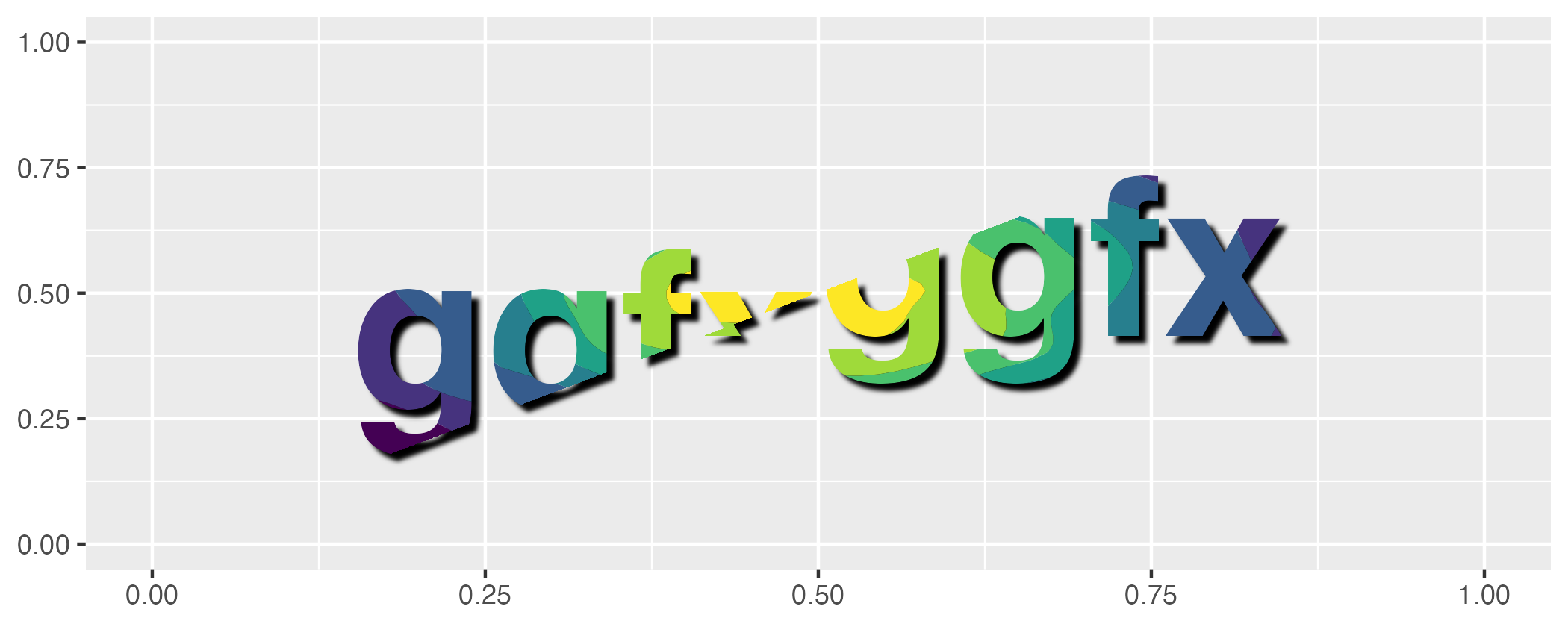ggfx is a (currently experimantal) package that allows the use of various filters and shaders on ggplot2 layers.

## Installation

You can install ggfx from CRAN in the usual manner (install.packages('ggfx')) or you can grab the development version directly from github using the devtools package:

# install.packages('devtools')
devtools::install_github('thomasp85/ggfx')

## Example

The basic API of ggfx is to provide a range of with_*() modifier functions instead of special versions of common geoms. This means that ggfx will work with any geom from ggplot2 and the extension packages (I think…). An example showing some of the different functionalities are given below. Note that the output is produced with regular geoms.

library(ggplot2)
library(ggfx)
ggplot() +
as_reference(
geom_polygon(aes(c(0, 1, 1), c(0, 0, 1)), colour = NA, fill = 'magenta'),
id = "displace_map"
) +
with_displacement(
geom_text(aes(0.5, 0.5, label = 'ggfx-ggfx'), size = 25, fontface = 'bold'),
x_map = ch_red("displace_map"),
y_map = ch_blue("displace_map"),
x_scale = unit(0.025, 'npc'),
id = "text"
) +
with_blend(
geom_density_2d_filled(aes(rnorm(1e4, 0.5, 0.2), rnorm(1e4, 0.5, 0.2)),
show.legend = FALSE),
bg_layer = "text",
blend_type = "in",
id = "blended"
) +
with_shadow("blended", sigma = 3) +
coord_cartesian(xlim = c(0, 1), ylim = c(0, 1), clip = 'off') +
labs(x = NULL, y = NULL)## Code of Conduct

Please note that the ggfx project is released with a Contributor Code of Conduct. By contributing to this project, you agree to abide by its terms.